# Get Day Name From a Date – Excel & Google Sheets

This tutorial will demonstrate how to get the name of the day from a date in Excel and Google Sheets.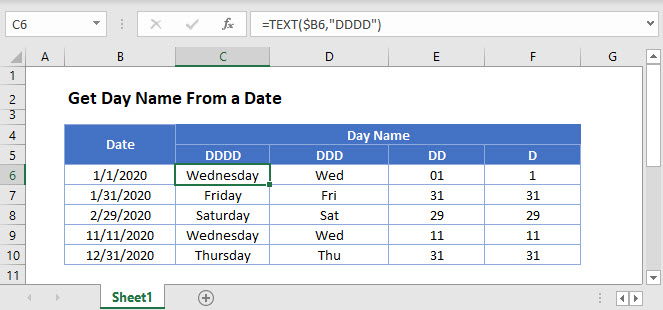## Extract the Day Name From a Date

You may need to extract the day name from a date value in Excel. We can do this with the TEXT Function.

The TEXT function converts values to text in the indicated format. You can choose whether you want to display the day name in a long (e.g. “Wednesday”) or short (e.g. “Mon”) form, or as a one- or two-digit number.

``=TEXT(B3,"dddd")``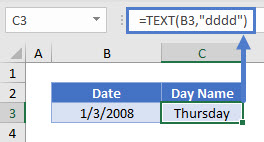``=TEXT(B3,"ddd")``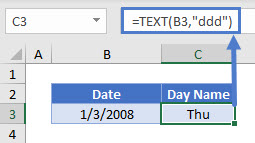In each of the previous two examples, the formula gives us the day of the week as a string of text. In each of the next two examples, the formula gives us the day of the month. The output is a number stored as text.

``=TEXT(B3,"dd")``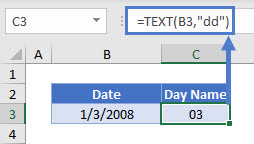``=TEXT(B3,"d")``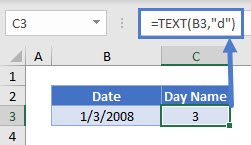Please note that when using the TEXT function, the original date is lost in the conversion; you only keep the text for the day name or number.

## Display the Day Name for a Date

There is another way to display the day name for a date: simply format the cell using a custom date format. Select the date, then go to Format Cells > Custom, and enter one of the above formats (dddd, ddd, dd, or d).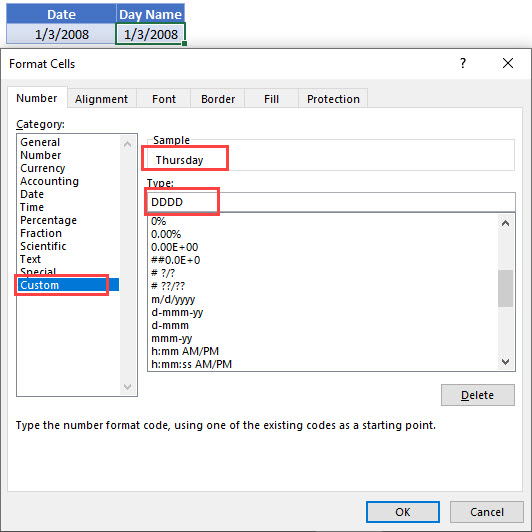## Get Day Name From a Date in Google Sheets

These formulas work exactly the same in Google Sheets as in Excel.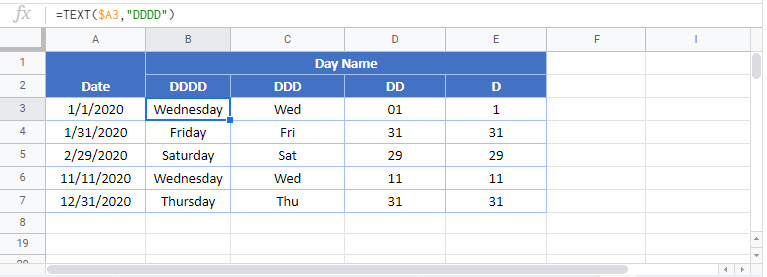### Excel Practice Worksheet

Practice Excel functions and formulas with our 100% free practice worksheets!